# thesIt

•#### Arif 12:41:37 pm on August 22, 2010 | 0 | # | Tags: cross-validation, error, estimation, k-fold, Michaelsen 1987, reference, Stone 1974

Stone 1974 is referenced in:
Michaelsen J. 1987. Cross-validation in statistical climate forecast models. J Climate Applied Meteorology, 26:1589-1600

1520-0450(1987)026-1589-cviscf-2.0.co;2.pdf

Set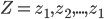$Z = z_{1}, z_{2},... , z_{1}$ consists of predictions and targets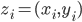$z_{i}=(x_{i}, y_{i})$

A set of prediction rule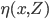$\eta (x,Z)$ will be used to predict y0 from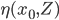$\eta (x_{0},Z)$

Let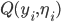$Q(y_{i},\eta_{i})$ be the accuracy.
by least squares this will usually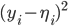$(y_{i}-\eta_{i})^{2}$
in other words expected Err is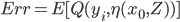$Err= E[Q(y_{i},\eta(x_{0},Z))]$

## MSE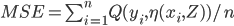$MSE= \sum_{i=1}^{n}Q(y_{i},\eta(x_{i},Z))/n$

In cross validation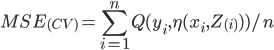$MSE_{(CV)}= \sum_{i=1}^{n}Q(y_{i},\eta(x_{i},Z_{(i)}))/n$

• #### Arif 06:29:08 pm on August 21, 2010 | 0 | # | Tags: cross-validation, Fu 1994, k-fold, validation

../sT-fold cross-validation (Stone 1974) repeats k times for a sample set randomly divided into k disjoint subsets, each time leaving one set out for testing and the others for training. Thus, we may call this technique “leave some out”

• #### Arif 12:14:08 pm on February 21, 2010 | 0 | # | Tags: cross-validation, faq, neural network

What are cross-validation and bootstrapping?

Cross-validation and bootstrapping are both methods for estimating generalization error based on “resampling”.

In k-fold cross-validation, you divide the data into k subsets of (approximately) equal size. You train the net k times, each time leaving out one of the subsets from training, but using only the omitted subset to compute whatever error criterion interests you. If k equals the sample size, this is called “leave-one-out” cross-validation.

• #### Arif 12:08:34 pm on February 21, 2010 | 0 | # | Tags: cross-validation, neural network, reference, Setiono 2001, verification

Feedforward Neural Network Construction Using Cross Validation. Rudy Setiono. Neural Computation 13(12): 2865-2877.

This article presents an algorithm that constructs feedforward neural networks with a single hidden layer for pattern classification. The algorithm starts with a small number of hidden units in the network and adds more hidden units as needed to improve the network’s predictive accuracy. To determine when to stop adding new hidden units, the algorithm makes use of a subset of the available training samples for cross validation. New hidden units are added to the network only if they improve the classification accuracy of the network on the training samples and on the cross-validation samples.

Extensive experimental results show that the algorithm is effective in obtaining networks with predictive accuracy rates that are better than those obtained by state-of-the-art decision tree methods.

10.1.1.112.9536.pdf